## Journal of Electrical Engineering and Electronic TechnologyISSN: 2325-9833

All submissions of the EM system will be redirected to Online Manuscript Submission System. Authors are requested to submit articles directly to Online Manuscript Submission System of respective journal.

Research Article, J Electr Eng Electron Technol Vol: 7 Issue: 2

# UHF Band-Pass Filter Based on Parallel Coupled Resonators

Mounir Belattar1*, Mohamed Lashab2 and Abdelaziz Benhabrou1

1Department of Electrical Engineering and Computer Engineering, University 20 Août 1955 University, Skikda, Algeria

2Department of Electrical Engineering and Computer Engineering, University Larbi Ben M’hidi, Oum EL Bouaghi, Algeria

*Corresponding Author : Mounir Belattar
Department of Electrical Engineering and Computer Engineering, University 20 Août 1955 University, Skikda, Algeria
Tel: 778626469
E-mail:
[email protected]

Received: June 04, 2018 Accepted: July 07, 2018 Published: July 14, 2018

Citation: Belattar M, Lashab M, Benhabrou A (2018) UHF Band-Pass Filter Based on Parallel Coupled Resonators. J Electr Eng Electron Technol 7:2. doi: 10.4172/2325-9833.1000163

## Abstract

The proposed microstrip filter is based on parallel coupled lines whose propagation mode is quasi-TEM, this type of filter take an important part in various communication systems. In this work we will study the procedure of design and realization of an UHF band-pass filter with centre frequency of 868.5 MHz and bandwidth of 1 MHz based on Coupled parallel lines, the obtained theoretical results are in good agreement with the literature.

## Introduction

In very high frequencies, inductors and capacitors lose their intrinsic characteristics. In addition, a limited range of component values is available from manufacturers. Therefore, for these frequencies, the passive filters are generally made using the distributed elements such as transmission line sections. Many works have been based on the use of waveguides for the design of filters [1,2]. However, waveguide systems are cumbersome and costly and of low power, compared to those designed from planar structures. In this work we are going to calculate the elements of a UHF micro ribbon filter with 868.5 MHz bandwidth 1 MHZ center frequency resonators, serving UHF transmitters to filter signals from a very close network that The GSM network, whose central frequency is 890 MHz.

## Filter Elements Calculation

Filter order

For a central frequency filter f0 equal to 868.5 MHz and 1 MHz bandwidth, we obtain: by transposing the prototype of a bandpass filter to that of a low pass filter. The components values can be calculated as follows.

g0 =1 (1)(2)(3)(4)(5)

Where bandpass filter parameters of order n=2 are given in Table 1.

n
0 1.0000
1 1.4029
2 0.7071
3 1.9841

Table 1: Bandpass filter parameter of order n=2.

We use the following equations for the design of the coupled parallel filter [1,2].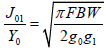(6)(7)(8)

Where g0, g1 ... gn are the elements of a prototype of a low pass filter with normalized cut-off frequency Ωc=1, and FBW is the passband of the bandpass filter. Jj, j + 1 are the characteristic admittances of J-inverters and Y0 is the characteristic admittance of the termination lines.

Odd and even characteristic impedances

The characteristic impedances of the even and odd modes of the coupled microstrip line resonators are determined according to [3,4] by: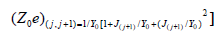(9)(10)

All these impedances are given in Table 2.

n Value 1 Value 2
0 51.8597 48.2692
1 50.0910 49.9094
2 51.8597 48.2692

Table 2: Odd and even characteristic impedances.

Calculation of the ratios W/h even and W/h odd

To present the odd and odd modes of the desired impedances, we determine the ratios of the simple equivalent microstrip lines (W/h) s, which are responsible for connecting the coupled line relationships to single-line relationships for a single microstrip line (Figure 1) .

Figure 1: Cross-section of a coupled symmetrical microstrip structure.(11)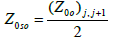(12)

Thus, the requires resonator:

For a single microstrip line, the approximate expressions for W/h in terms of Zc and εr, drawn by Hammerstad  are given as follows:(13)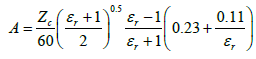(14)

For W/h ≥ 2(15)(16)

Calculation of W/h and S/h ratios

At this point he is able to find (W/h) se and (W/h) so, by applying Z0se and Z0so (as Zc) to the equations of simple microstrip lines . Now, the ratios w/h and s/h can be expressed for the desired coupled microstrip line using a set of approximate equations (4,7):(17)(18)

Calculation of the microstrip length

For each pair of coupled quarter wave sections, the guided wavelength of the quasi-TEM microstrip mode can be given by:(19)

Thus, the required resonator:(20)

The expressions giving better precision  are as follows:

For broad microstrip lines, where W/h ≥ 1(21)

For narrow microstrip lines, where W/h ≤ 1(22)

## Accurate Method for Calculating Filter Parameters

The static microstrip line model gives accurate results for low frequencies (up to about 868.5 MHz). It uses physical dimensions of the microstrip line as normalized parameters .(23)

In n homogeneous medium. The equations here use air, as a homogeneous material. The impedance is given by:(24)(25)

The effective shielded impedance represented by the expression:(26)

In order to take into account the shielding, effects, a fill factor q is introduced : The effective relative permittivity is therefore given by:(27)

The characteristic impedance of the microstrip line placed on a dielectric with relative permittivity εr is given by:(28)

Dispersive model of coupled parallel microstrip lines

A symmetric pair of coupled microstrip lines is composed of two parallel lines of equal widths W with a spacing S and of length L mounted on a non-magnetic dielectric substrate of thickness h (Tables 3 and 4). And having a permittivity relate εr

Duroid
W1 2.6120 S1 0.6813
W2 2.6292 S2 2.1700
W3 2.6120 S3 0.6813

Table 3: Widths W and separation S of the lines filter.

Duroid (mm)
L1 41.2640
L2 41.3411
L3 41.2640

Table 4: Length of value of parallel lines.

The equations expressing the effective permittivity are given by the following expressions:(29)(30)

Where fn GHz.mm is the normalized frequency with respect to the substrate height, the effective characteristic impedance due to the dispersion is given by Jansen and Kirschning :(31)

Evaluation of characteristic impedances and effective relative permittivity

The physical dimensions of the structure were again standardized with respect to the substrate height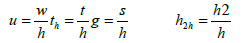(32)

The results are accurate to 1 percent in the range:(33)

Where εr is the relative permittivity of the substrate material for the same static mode (f=0) whereas the effective permittivity is calculated according to Hammerstad  as follows:(34)

Kirschning and Jansen  have reshaped the equations Hammerstad and Jensen for the odd mode of static permittivity, in order to improve the accuracy, to obtain: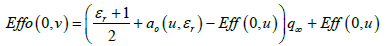(35)

The term odd-shielding correction is expressed as: The quantity εeff (0) is the effective permittivity of a single microstrip of thickness w and the relative effective permittivity in the case of a zero-width conductor is calculated. The indices "e" and "o" designate the even and odd modes, respectively, and the argument 0 implies static parameters. For the even mode, the characteristic impedance is expressed as:(36)

The shielding effects are again counted, where the characteristic impedances of the even and odd modes of a symmetric pair of microstrip lines coupled in a homogeneous air dielectric (εr=1) are calculated and the ΔZ0 correction term (0) is computed from another set of expressions for even and odd modes. Whereas, for the same modes, the correction is expressed by:(37)

And for the odd mode the correction is given by: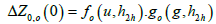(38)(39)

In order to obtain the corrections made on the impedances of the coupled lines on a dielectric substrate different from air, the corrections have been obtained in the case of air; this must be divided by the square root of the effective permittivity:(40)

And(41)

The impedance dispersion in the even mode is obtained from the expression(42)

Synthesis of coupled microstrip lines

In the synthesis procedure, Ze and Zo for the coupled lines are known and it is necessary to find W/h and S/h. The first step is to find the two single line shape ratios (w/h)se and (w/h)so corresponding to the impedance Ze/2 and Zo/2, Wheeler's theory  provides curves , for obtaining the ratios graphically. The W/h, and S/h ratios for the coupled lines are now solved simultaneously by the following formulas (Figure 2):

For εr<6(43)(44)

For εr >6(45)

Such as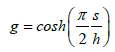(46)(47)

## Results

After all the calculations, we arrive at the design of the filter UHF of order 2 (Figure 3 and Table 5) with a substrate Duroid of height h=0.79 mm and of relative permittivity εr=2.35, operating at the central frequency f0=868.5 MHz with 1 MHZ bandwidth.

Dimensions Effe     Z0
S/h W/h Odd Even Odd Even
0.1 2.25 1.7590 2.0448 36.4813 73.6391
3.0 1.7916 2.0825 32.2078 59. 4291
4.0 1.8320 2.1196 28.0135 47. 3662
0.3 2.25 1.7795 2.0484 43.1014 71. 4389
3.0 1.8150 2.0852 37.2333 57. 9618
4.0 1.8572 2.1216 31.7039 46. 4085
0.5 2.25 1.7953 2.0497 46.6770 69. 7295
3.0 1.8320 2.0859 39.8780 56. 8252
4.0 1.8748 2.1219 33.5922 45. 6746
0.7 2.25 1.8086 2.0493 49.0745 68.3330
3.0 1.8459 2.0853 41.6310 55.8874
4.0 1.8886 2.1210 34.8284 45.0653
0.9 2.25 1.8202 2.0479 50.8125 67.1692
3.0 1.8576 2.0836 42.8968 55.0955
4.0 1.9001 2.1193 35.7173 44.5453

Table 5: Characteristic impedances and effective relative permittivity’s and dimensions computed for a frequency f0=868.5 MHz in even and odd mode.

ZL (f) is the dependent frequency of powering up a single microstrip with a characteristic impedance width W This calculation gives the impedances of the even and odd modes as well as the even and odd efficient dielectric constants of the microstrip coupled lines on a Duroid substrate illustrated in Figure 4. Figure 5 shows the variations of the ratio W/h as a function of S/h in the even odd coupling modes of the microstrip line coupled for the Duroid case.

Figure 5: Odd and Even characteristic impedance of microstrip coupled as a function of frequency.

## Conclusion

In this work, one of our objectives was to present the design procedure of a Tchebychev-type microstrip UHF bandpass filter based on parallel coupled lines. The design of these filters requires the determination of widths Wi of coupled parallel micro-line lines, the widths li of the resonators as well as the Si distances separating these lines. In this context, a calculation program has been developed to calculate these elements accurately.